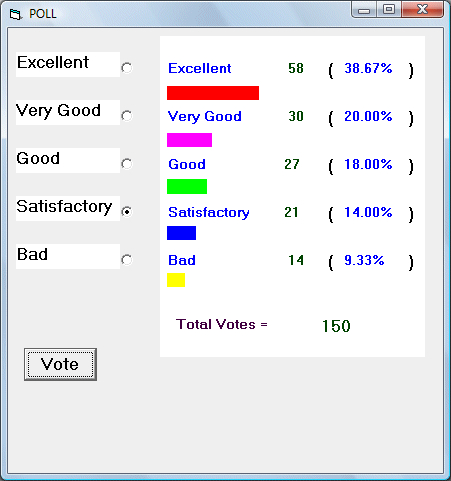# Polling System

Survey and polling tools are often used in marketing or politics to assess ratings for certain services or products. Polling tools can take many forms, some just use a simple dichotomous scale of Yes and No, or a more complex Likert Scale that consists of three or more choices. You can create Polling tool in Visual Basic easily by using the option buttons. In our program, the users are given five choices, Excellent, Very Good, Good, Satisfactory and Bad.The results are presented in frequency and percentage respectively.

In this example, we include a graphical display of the percentages of the five scores using the Line method. The syntax to draw the rectangular bar in a picture box is

Picture1.Line (x1, y1)-(x2, y2), color, BF

where (x1,y1) is the coordinates of the upper left corner of the bar and

(x2,y2) is the coordinates of the lower right corner of the bar.

To show the bar length according to the percentage, we can use certain value to multiply the decimal value of each score and put it under x2.

Finally, we can use the Picture1.Cls method to clear the picture box in order to refresh the drawing.

### The Code

Dim total, Excel_total, VG_total, G_total, Sat_total, Bad_total As Integer
Dim Excel_percent, VG_percent, G_percent, Sat_percent, Bad_percent As Single
Dim done As Boolean

Private Sub cmd_Vote_Click()
Picture1.Cls

If Option_Excel.Value = True Then
Excel_total = Excel_total + 1
Lbl_ExcelTotal = Excel_total
ElseIf Option_VG.Value = True Then
VG_total = VG_total + 1
Lbl_VGTotal = VG_total
ElseIf Option_G.Value = True Then
G_total = G_total + 1
Lbl_GTotal = G_total
ElseIf Option_Sat.Value = True Then
Sat_total = Sat_total + 1
Lbl_SatTotal = Sat_total
End If
total = Excel_total + VG_total + G_total + Sat_total + Bad_total

Lbl_Total = total

Excel_percent = Excel_total / total
VG_percent = VG_total / total
G_percent = G_total / total
Sat_percent = Sat_total / total
Lbl_Excel.Caption = Format(Excel_percent, "Percent")
Lbl_VG.Caption = Format(VG_percent, "Percent")
Lbl_G.Caption = Format(G_percent, "Percent")
Lbl_Sat.Caption = Format(Sat_percent, "Percent")
Picture1.Line (100, 750)-(3800 * Excel_percent, 950), vbRed, BF
Picture1.Line (100, 1450)-(3800 * VG_percent, 1650), vbMagenta, BF
Picture1.Line (100, 2150)-(3800 * G_percent, 2350), vbGreen, BF
Picture1.Line (100, 2850)-(3800 * Sat_percent, 3050), vbBlue, BF
Picture1.Line (100, 3550)-(3800 * Bad_percent, 3750), vbYellow, BF

End Sub
<

### The Interface